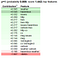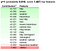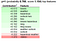# NLP for Disaster Tweets Detection

One of the powers of machine learning is to use it for classifying data or even predicting any event. This time we will play with text data. So, we have tweet data with keyword, location, text, and label. The labels are either 1 or 0 which shows if the tweets contain any information about the disaster (label ‘1’) or not (label ‘0’). Some of the data does not have any values on keyword or location so we have to do something to handle that problem.

You can find the dataset in the Github and more explanation (In Indonesian) in YouTube link.

Okay, let’s start.

1. Import all the packages we need
`import pandas as pdimport numpy as npimport seaborn as snsimport matplotlib.pyplot as pltimport reimport stringimport eli5from string import punctuationfrom collections import defaultdictfrom nltk import FreqDistfrom sklearn.linear_model import LogisticRegressionfrom sklearn.pipeline import make_pipelinefrom sklearn.feature_extraction.text import CountVectorizer`

2. Set up the directory and call the train, test, and ground truth files

`directory = r'C:\Users\LENOVO\Jupyter Notebook\Real or Not NLP with Disaster Tweets'train = pd.read_csv(directory+'\\train.csv')test = pd.read_csv(directory+'\\test.csv')gt = pd.read_csv(directory+'\\submission.csv')`

3. Before we do the main task (which creates a model), it’s better to know how our data looks like. This step is important because it will show us how our data looks like. From here, we can take any action of what model we should use and what preprocessing we need.

4. we can see the distribution of our data for class ‘0’ and ‘1’ by this code below. Well, the graphic shows we have more class ‘0’ data than class ‘1’. Probably the difference is only about 1200 sentences. Okay now we know there are only 2 classes, so this task is a binary classification task. What model is best known to handle binary classification task? One of them is Logistic Regression, so we are going to use it in our experiment.

`x=train.target.value_counts()sns.barplot(x.index,x)plt.gca().set_ylabel('samples')`

5. Now, let’s see how many characters contains in every tweet. Good, they are not so different. Probably 125–140 characters are common in every tweet.

`fig, (ax1, ax2) = plt.subplots(1, 2, figsize=(10,5))tweet_len = train[train['target']==1]['text'].str.len()ax1.hist(tweet_len, color='red')ax1.set_title('Disaster tweets')tweet_len = train[train['target']==0]['text'].str.len()ax2.hist(tweet_len, color='green')ax2.set_title('Not disaster tweets')fig.suptitle('Characters in tweets')plt.show()`

6. Now let’s see how many words in every tweet are. Seems like they are similar, except for the tweets with 13–17 words.

`fig, (ax1, ax2) = plt.subplots(1, 2, figsize=(10,5))num_words = train[train['target']==1]['text'].str.split().map(lambda x: len(x))ax1.hist(num_words, color='red')ax1.set_title('Disaster tweets')num_words = train[train['target']==0]['text'].str.split().map(lambda x: len(x))ax2.hist(num_words, color='green')ax2.set_title('Non disaster tweets')fig.suptitle('Words in a tweets')plt.show()`

7. Next, let’s see average word length in every tweet. Great, the distributions are similar which is about 4–6 characters in a tweet. Which mean, we don’t have to worry about doing any preprocessing to the character.

`fig, (ax1, ax2) = plt.subplots(1, 2, figsize=(10,5))word = train[train['target']==1]['text'].str.split().apply(lambda x: [len(i) for i in x])sns.distplot(word.map(lambda x: np.mean(x)), ax=ax1, color='red')ax1.set_title('Disaster tweets')word = train[train['target']==0]['text'].str.split().apply(lambda x: [len(i) for i in x])sns.distplot(word.map(lambda x: np.mean(x)), ax=ax2, color='green')ax2.set_title('Non disaster tweets')fig.suptitle('Average word length in each tweet')`

8. It is also important to know what are the common words in each class. This result will tell us whether we should do any stopword filtering or not. There are a lot of punctuation, probably comes from emoticon. Then, we will need to see whether if remove it will give better result or not.

`text_disaster = train[train['target']==1]['text'].str.split()text_Nodisaster = train[train['target']==0]['text'].str.split()fdist = FreqDist(word.lower() for sentence in text_disaster for word in sentence)fdist.plot(10, title="Disaster tweets")dic=defaultdict(int)punct = [fdist[p] for p in punctuation]plt.figure(figsize=(12, 6))sns.barplot(punct, list(punctuation))fdist = FreqDist(word.lower() for sentence in text_Nodisaster for word in sentence)fdist.plot(10, title="Non disaster tweets")dic=defaultdict(int)punct = [fdist[p] for p in punctuation]plt.figure(figsize=(12, 6))sns.barplot(punct, list(punctuation))`

9. Done with 1-gram, it’s also important to check the most common bi-gram in the tweet. Hmm, seems like most of them coming from URL, which is not good. So I suggest, we should remove all URLs from the tweet.

`def get_top_tweet_bigram(corpus, n=None):    vec = CountVectorizer(ngram_range=(2, 2)).fit(corpus)    bag_of_words = vec.transform(corpus)    sum_words = bag_of_words.sum(axis=0)     words_freq = [(word, sum_words[0, idx]) for word, idx in vec.vocabulary_.items()]        words_freq =sorted(words_freq, key = lambda x: x, reverse=True)    return words_freq[:n]plt.figure(figsize=(10,5))top_tweet_bigram = get_top_tweet_bigram(train['text'].tolist())[:10]x,y = map(list, zip(*top_tweet_bigram))sns.barplot(y,x)`

10. Okay, we’ve done with the analysis. Now it’s time to do some cleaning.

11. Do the basic one, fill the empty value. Fill the empty value by any random string probably will make our data messier. So it is better to fill it with a period (.)

`train = train.fillna('.')test = test.fillna('.')`

12. Create a function for remove every URL

`def remove_URL(text):    url = re.compile(r'https?://\S+|www\.\S+')    return url.sub(r'',text)`

14. From our analysis in point 8, we will make a function to remove any emoji. You can add any emoji code as you wish

`def remove_emoji(text):    emoji_pattern = re.compile("["                           u"\U0001F600-\U0001F64F"  # emoticons                           u"\U0001F300-\U0001F5FF"  # symbols & pictographs                           u"\U0001F680-\U0001F6FF"  # transport & map symbols                           u"\U0001F1E0-\U0001F1FF"  # flags (iOS)                           u"\U00002702-\U000027B0"                           u"\U000024C2-\U0001F251"                           "]+", flags=re.UNICODE)    return emoji_pattern.sub(r'', text)`

15. Sometimes, removing punctuation can give a better result to the model. So, let’s try

`def remove_punct(text):    table=str.maketrans('','',string.punctuation)    return text.translate(table)`

16. One thing for sure, all of these are just assumption. Which mean we are not sure yet whether remove URL, emoji, and punctuation can improve our result or not. Maybe it will make the model perform worse. Therefore, we will try some combination when using it. This function below will help us to do that.

`def preprocessing(re_URL=False, re_emoji=False, re_punct=False):    data_train = train['keyword'] +' '+ train['location'] +' '+ train['text']    data_test = test['keyword'] +' '+ test['location'] +' '+ test['text']if re_URL:        data_train = data_train.apply(lambda x : remove_URL(x))        data_test = data_test.apply(lambda x : remove_URL(x))        print("URL Removed")    if re_emoji:        data_train = data_train.apply(lambda x : remove_emoji(x))        data_test = data_test.apply(lambda x : remove_emoji(x))        print("Emoji Removed")    if re_punct:        data_train = data_train.apply(lambda x : remove_punct(x))             data_test = data_test.apply(lambda x : remove_punct(x))        print("Punctuation Removed")    return data_train, data_test`

17. All done! Now let’s start training. To make it easy, let’s just put the vector and classifier inside a pipe. This a good function provide by sklearn.

`def fit_and_predict(vec, clf, X_train, y_train):    pipe = make_pipeline(vec, clf)    pipe.fit(X_train, y_train)        y_test = gt['target'].tolist()    acc = pipe.score(X_test, y_test)    print("Accuracy: ", acc)`

18. Let’s try our baseline, online using 2-gram countvector. The result is not bad

`train['sums'] = train['keyword'] +' '+ train['location'] +' '+ train['text']test['sums'] = test['keyword'] +' '+ test['location'] +' '+ test['text']X_train = train['sums'].tolist()y_train = train['target'].tolist()X_test = test['sums'].tolist()vec = CountVectorizer(ngram_range=(1,2))clf = LogisticRegression()fit_and_predict(vec, clf, X_train, y_train)#Accuracy:  0.7955868832362857`

19. Now try add stopword to the baseline and lowercase all letters. Good! It goes to 80% now

`train['sums'] = train['keyword'] +' '+ train['location'] +' '+ train['text']test['sums'] = test['keyword'] +' '+ test['location'] +' '+ test['text']X_train = train['sums'].tolist()y_train = train['target'].tolist()X_test = test['sums'].tolist()vec = CountVectorizer(ngram_range=(1,2), lowercase=True, stop_words='english')clf = LogisticRegression()fit_and_predict(vec, clf, X_train, y_train)#Accuracy:  0.8035550107263255`

20. Next, let’s try to apply our assumption from the analysis. We remove URL, emoji, and punctuation. Hmm, okay it’s worse

`train['sums'], test['sums'] = preprocessing(re_URL=True, re_emoji=True, re_punct=True)X_train = train['sums'].tolist()y_train = train['target'].tolist()X_test = test['sums'].tolist()vec = CountVectorizer(ngram_range=(1,2), lowercase=True, stop_words='english')clf = LogisticRegression()fit_and_predict(vec, clf, X_train, y_train)#Accuracy:  0.7971192154459087`

21. Maybe the emoji is still important because it can describe sentiment information. So let’s try to still use it.

`train['sums'], test['sums'] = preprocessing(re_URL=True, re_emoji=False, re_punct=True)X_train = train['sums'].tolist()y_train = train['target'].tolist()X_test = test['sums'].tolist()vec = CountVectorizer(ngram_range=(1,2), lowercase=True, stop_words='english')clf = LogisticRegression()fit_and_predict(vec, clf, X_train, y_train)#Accuracy:  0.7971192154459087`

22. Hmm okay, the result still worse. How if we just remove punctuation? Well the result still worse than the result on step 19

`train['sums'], test['sums'] = preprocessing(re_URL=False, re_emoji=False, re_punct=True)X_train = train['sums'].tolist()y_train = train['target'].tolist()X_test = test['sums'].tolist()vec = CountVectorizer(ngram_range=(1,2), lowercase=True, stop_words='english')clf = LogisticRegression()fit_and_predict(vec, clf, X_train, y_train)#Accuracy:  0.796812749003984`

23. Why those things happened? Let’s try to figure it out. Let’s see what are the top-10 features on our baseline experiment. Prediction on the first row and last 2nd row of testing data. I believe the table is pretty self-explanatory, you can see what features are important and it’s weight. Also, you can see prediction result on words level by using show_prediction function.

`eli5.show_weights(clf, vec=vec, top=10)eli5.show_prediction(clf, X_test, vec=vec, target_names=['0', '1'])eli5.show_prediction(clf, X_test[-2], vec=vec, target_names=['0', '1'])`Top features, prediction of data and prediction of data[-2] of baseline model

24. Next, we gonna see what are the top-10 features in our experiment on step 19. Prediction on the first row and last 2nd row of testing dataTop features, prediction of data and prediction of data[-2] of model from step 19

25. Now we gonna see what are the top-10 features in our experiment on step 20. Prediction on the first row and last 2nd row of testing dataTop features, prediction of data and prediction of data[-2] of model from step 20

Written by